# Dividing a sphere into twelve "identical" shapes.

## Recommended Posts

Found another interesting figure. When rolling clay between your palms to make a sphere you keep "turning" the ball to flatten the high sides. If you don't turn the clay and just keep the palms going in that circular motion, you wind up with two flat cones attached at their bases. Dividing each of the cones into three sections opposite the three sections of the other cone, and making a thumb print in the center of each section as a guide, you can flatten each side with a thumb print at its center, and you wind up with a monoclinique or a parallelepiped or something or rather that is a cross between a cube and hexagonal figure, once you mark it into the 12 sections with the internal 90 degree and 120 degree angles.

I call it a squex (cross between a squished cube and a hexagonal figure) but I am sure it has a real name. Still like the thought because it is so close to both a cube and to the two connected cones, that one can see the relationship between the two dimensionally flat surface of a cone and the three dimensionally flat surface of a sphere. And the diamond shape shows its relationship to the square when the surface is tilted.

Regards, TAR

##### Share on other sites

• 2 weeks later...
• Replies 184
• Created

#### Popular Days

If you make a great circle on a sphere in red, and then make a hole somewhere on this circle and through the hole make another great circle 60 degrees (120) offset, in yellow, you get the two lines crossing each other twice, making two Xs on the sphere. Halfway between the 120 degree sides of the Xs, make another cross of a blue and green great circle with a similar X having the 120 degree sides of the Xs oriented toward each other. You will have just inscribed a spherical cuboctohedron on the sphere, with 6 square areas and 8 triangular areas evident.

Each of the four colored lines, represent the plane of each of the four intersecting hexagonal planes we have been taking about in the dual Cuboctahedron/Spherical Rhombic Dodecahedron. If you wander 30 degrees NEish from the hole, along the red line and make another great circle in red intesecting the first red line at a 90 degree angle and repeat this 60 degrees along the red line later, and then again in another 60 degrees the three new red great circles will divide the sphere into 6 "orange" slices, each divided in half by the first red great circle.

Attempting this placement of new yellow great circles at 90 degrees to the 30 degrees from the hole mark. 90 degrees from the hole and 150 degrees from the hole mark along the first yellow great circle, one finds the first new great circle can be marked in yellow, the second new great circle is already present in red and the third new great circle can be marked in yellow.

Attempting marking the blue great circle with three evenly spaced great circles in the same manner as before one finds the first great circle already present and marked in yellow, the second great circle can be marked in blue, and the third great circle is already present and marked in red.

Attempting to mark the green great circle into its six segments with the three great circles dividing the sphere into six "orange" slices, one finds the new great circles already placed and marked in yellow, blue and red respectively.

Figure shown here is the result of following this procedure, with black diamonds inscribed on the inside of certain of the great circles, indicating where the 12 diamonds of the Spherical Rhombic Dodecahedron fall.

##### Share on other sites

• 3 weeks later...

Here is another squex, or sqube with the six sides. When you divide it up into the 12 sections though, the 12 sections are not identical. There are 6 of the 12 that are identical which you can see as the three on the top making a triangle out of three shield (kite) shapes and three on the bottom. The 6 around the edges of the hexagon are of two types. The kite shape can imaginarily be cut in half along the long axis yielding two right triangles which you can flip and join together along the long axis to make a rectangle. This is the shape of the 6 segments around the perimeter of the hexagon. 3 are left handed and three are right handed. This is hard to explain but you can see how they alternate.

Interesting that the shield shape works like this.

You can still follow six segments around on a plane, and there is still four such planes, such as is the case with all the figures so far divided into the 12 segments.

Regards, TAR

##### Share on other sites

• 2 weeks later...

Here I have divided the cube, truncated octahedron, spherical rhombic dodecahedron, tetrahedron, octahedron and cuboctahedron into the 12 identical sections.

I put 6 red, 6 yellow, 6 blue and 6 green toothpicks into the center of each section in each figure each in the pattern described with the red and yellow plane
intersecting in section 1 and the blue and green intersecting in section 11...etc. Anyway, all seven figures have the same pairs of planes intersecting in the
same manner. The figure in the middle is the spherical rhombic dodecahedron surrounded by the spherical framework of a cuboctahedron.
The four pictures of the same collection are taken normal to the red, yellow, blue and green planes, respectively.

Regards, TAR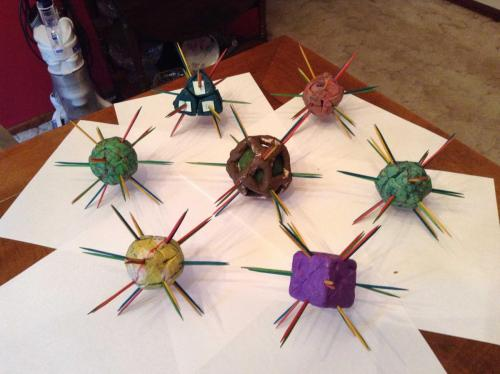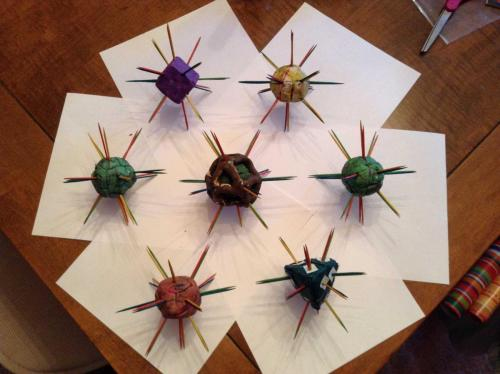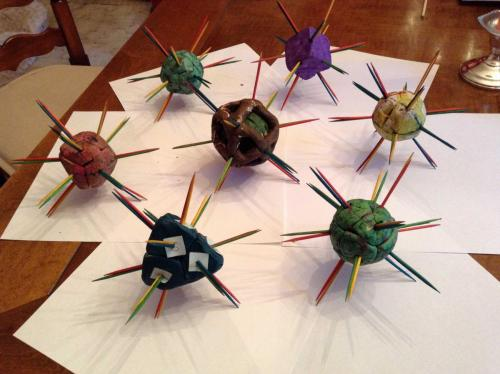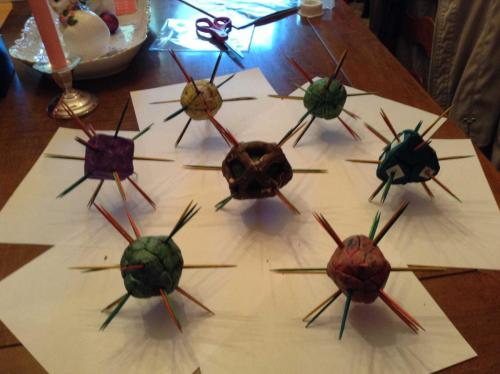##### Share on other sites

actually the way they uploaded, it's

Blue Green

Red Yellow

##### Share on other sites

• 2 weeks later...

Made a little progress today toward translating known coordinate systems into TAR coordinates. Here is the convention for lining up Cartesian coordinates with the Earth in terms of the 12 segments of the sphere.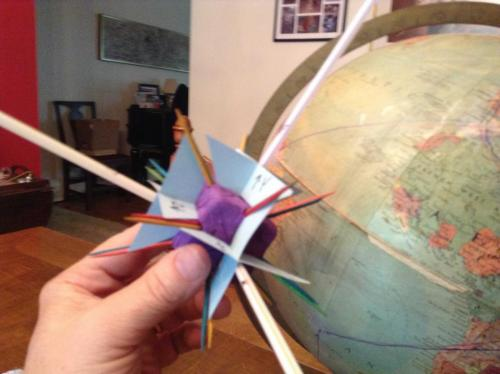We are putting the center of segment 1 on the Prime Meridian, where it intersects the equator. This "direction", the center of segment one, is exactly R 0(360) degrees/ Y 0(360) degrees, which conventionally can be lined up with the line x=z. The pairs of toothpicks shown in the previous post happen to coordinate exactly with the lines

(goofy equations removed...under revision)

These lines (the double toothpicks) go exactly through the middle of each edge of the cube.

If we let the axis of the Earth be the y axis and run the x axis through Africa (a point on the equator between Kenya and the Belgian Congo on my globe), the negative x axis would come through the surface on the equator, between Hawaii and the Marquesas Islands. The positive z axis would break the surface on the equator in the north of Brazil , the negative z on the equator between Australia and the Philippines.

The association in regards to the center of the twelve TAR sections would be

(numbers in degrees)

1 R0(360)/Y0(360) is (to be determined)

2 R60/G60 is (to be determined)

3 B60/r120 is (to be determined)

4 ye180/r180 is (to be determined)
5 g240/r240 is (to be determined)

6 R300/b240 is (to be determined)

7 g120/Y60 is (to be determined)

8 ye120/b120 is (to be determined)

9 ye240/G300 is (to be determined)

10 B300/Y300 is (to be determined)

11 B0(360)/G0(360) is (to be determined)

12 g180/b180 is (to be determined)

I am thinking, with the center of the RY section, being an arbitrary/conventional point on the equator, and the North pole being where sections 7,2,3 and 8 touch, any spherical system, where an axis of rotation, an up, and a choosen point on the equator can be decided upon, can be designated in TAR coordinates, and thusly a transform from any spherical coordinate system to TAR coordinates and back again, should be possible.

Regards, TAR

Please note two errors in drawing diamond 1 on the globe. The top of the diamond is slightly to the West of the Prime Meridian (is lined up with Scotland) and the bottom of the diamond, is WAY off, following a line where the globe was glued, that has nothing to do with anything.

Edited by tar
##### Share on other sites

1 R0(360)/Y0(360) is is the line where z=x

2 R60/G60 is the line where y=x

3 B60/r120 is the line where y=-z

4 ye180/r180 is the line where -z=-x
5 g240/r240 is the line where -y=-x

6 R300/b240 is the line where -y=z

7 g120/Y60 is the line where y=z

8 ye120/b120 is the line where y=-x

9 ye240/G300 is the line where -y=-z

10 B300/Y300 is the line where -y=x

11 B0(360)/G0(360) is the line where -z=x

12 g180/b180 is the line where z=-x

Edited by tar
##### Share on other sites

"Lines" here are radial lines from the center of the figure under discussion (ie. sphere, cube, tetrahedron) and likewise are rays starting at the origin of an x,y,z Cartesian coordinate grid, made of cubic units.

Insight is, that the center of each of the twelve sections of the sphere, is in the same direction as the center of one of the edges of a cube and a cube can be exactly placed on a three axis right angle, coordinate grid. Thus providing translation points between the four intersecting hexagonal planes of the figures that divide nicely into the 12 identical sections (6 axes at 60 degrees to each other), and the x,y,z 3 axis, right angle grid we are already familiar with.

Regards, TAR

Edited by tar
##### Share on other sites

If you take six crosses of card stock, you can put them in the center of the six sides of the cube and describe the xy plane, the xz plane and the yz plane of the Cartesian coordinated system.

If you take each of the six crosses (made slightly longer to reach across the diagonal of a face) and orient them on the diagonals of the face, you no longer (keep the two card stock pieces orthogonal to each other, but rotate each of the pairs 45 degrees, to go from going across and up and down, from edge to edge, to going diagonally from corner to corner,) are describing the eight sections of space, but are describing the twelve sections of space, that are being considered in this thread. (the twelve "janus" sections of space)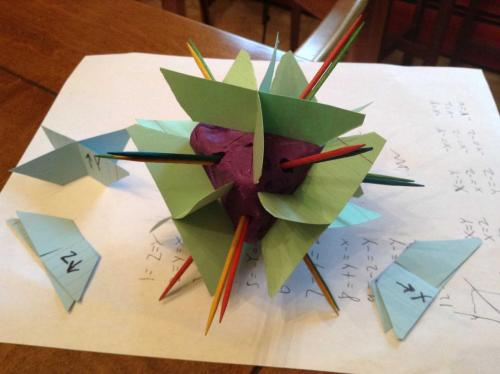Regards, TAR

##### Share on other sites

Here is a nice 2D layout of the 3D space we are talking about.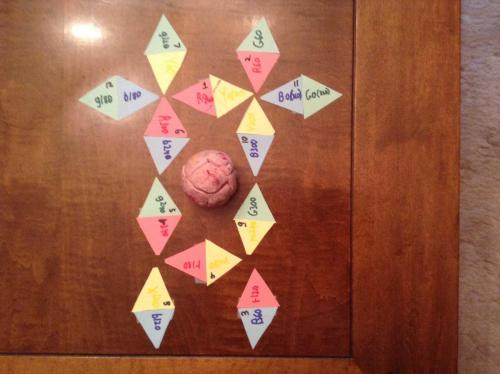Notice you can follow each of the 4 hexagonal planes around, 0,60,120,180,240,300,(360). The red in a left semicircle, the yellow in a right semicircle, the green in a circle and the blue in a broken circle (that completes if you take the two bottom diamonds and put them on top ((wrap around)).)

Regards, TAR

Still working on what the length of the line between vertices of the spherical rhombic dodecahedron must be, but I am thinking it is 1/6 the circumference based on the following assumption.

A hexagon is made of 6 equilateral triangles.

The spherical rhombic dodecahedron can be simulated with 24 equilateral triangles of cardstock (actually 48 taped and clipped together to simulate 24.)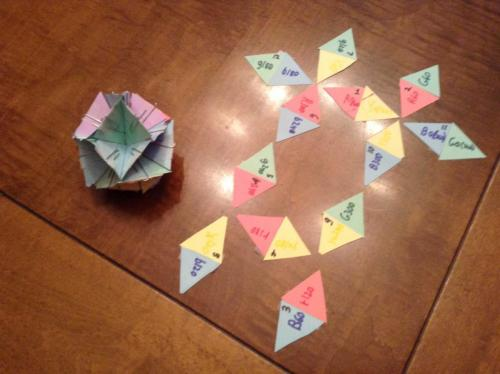If you imagine the top edge of each of the triangles (the edge normal to the center) as a chord of a circle circumscribing a hexagon, the vertices would have be one r away from each other, in a straight line, and likewise one 1/6 of a circumference.

Since circumference = pi D and the radius of our 1/4 pound is 60, 60th of an inch, then in 60th of an inch;

Circumference= pi * 120= approx 377 and 1/6 of that, is approx 62.8.

So, if my assumption is correct (that you can exactly make a spherical arrangement with equilateral triangles)(or that the flat sides of Janus sections are equilateral triangles with a 1/6 circumference ice cream scope on top of the cone) then;

chord=60

arc=62.8

(62.831853071795864769252867666559)

Regards, TAR

The equilateral triangles shown here are not to 1/4lb. of clay scale. The edges of the triangles here are approximately 100, 60th of an inch, or about 5/3 scale.

Actually about 105/60th of an inch to a side. My triangles were not very consistent. In making the triangles I incorrectly thought I could cut two large equilateral triangles out of a 3 x 5 card, with two left over pieces that would, if put together make a third large triangle, and I was cutting 4 equilateral triangles from each large triangle. Turns out, you cannot cut a 3 x 5 card up thusly. 60 degrees from corner to edge is 210, 60th of an inch. 60 degrees and 210 60th of an inch makes a nice equilateral, but from that point gets you close to the other corner, but you run out of card. Your first large triangle is 210 by 210 by 210. Your second triangle is not equilateral, instead is 210 by 200 by 192.

Edited by tar
##### Share on other sites

Except, my assumption might be incorrect. Still working on it.

##### Share on other sites

Looks like my assumption might be incorrect.

Taking measurements of the pink spherical rhombic dodecahedron, by "rolling" the 60th of an inch edge of a ruler along the short diagonal of the 12 diamonds, I got an average measurement of 68.5.

The long diagonals averaged about 90, whereas 16 measurements of the edges of the diamonds averaged 57.5.

If my assumption were to be correct, the short diagonal would have to be equal to 1/6 of a hexagon, and/or 1/6 of a circumference, since there are places on the figure where you can count 4 edges and two diagonals, tracing a great circle around the figure. By measurement this seems to be "not the case" as the edges averaged 57.5 and the short diagonals 68.5.

The measurements showed the divisions to be somewhat inconsistent, but close enough to expectations, to consider almost correct(the long diagonals should add up to 377 if we are measuring a 2 inch in diameter sphere, and 4 times 90 is 360 which is close, percentagewise to 377. ) 58 and 69 are not so close, and the length of the short diagonal of a diamond seems like it might be longer than a side of the diamond.

Still working on proving my assumption correct, or incorrect.

##### Share on other sites

Made the figure today from equilateral triangles. Made the framework for each Janus section, and taped them together. Each "wall" or boundary is two equilateral triangles of the same color with the degree around the hexagonal plane that the boundary represents in the TARadian coordinate system I came up with in December. This figure allows one to visulalize how the Red, Yellow, Blue and Green planes intersect one another and completely describe the sphere.

Going around the equator West to East.

(the equilateral triangles are 60 60th of an inch per side, which is also the radius of the 2 inch ball that is cut into the 12 Janus sections)

Regards, TAR

The hexagonal planes might not intersect each other at 60/120 degrees as I assumed before. Measuring the pink Spherical Rhombic Dodecaheron, the Xs look to be closer to 110/70 degrees.

Interesting but not necessarily the actual measurement, or pertinent, is the fact that 110/70 is 1.5714. Double that, is 3.1428.

Edited by tar
##### Share on other sites

• 4 weeks later...

Here is a really neat application of the Spherical Rhombic Dodecahedron combined with the grid system for TAR coordinates, repurposed and calibrated to be used as a one picture color space descriptor.

I came up with it earlier today and am calling it the HAS system (Hue, Additive Color, Subtractive Color) system.

It is similar to the HSL (hue, saturation, luminence) but uses the additive nature of light and the subtractive nature of pigments to move a hue toward white, or toward black.

The hue system is the same as the HSL system with Red at 0 degrees, Yellow at 60 degrees, Green at 120 degrees, Cyan at 180 degrees Blue at 240 degrees and Magenta at 300 degrees, with all the rainbow colors at the appropriate places between. These six diamonds are taking the place of diamonds 2,7,12,5,9, and 11.

Red Green and Blue are additive colors and the 12 segment figure works out perfectly to have a red diamond, a red yellow diamond and a red magenta diamond.

a green diamond a green cyan diamond and green yellow diamond.

a blue diamond, a blue magenta diamond, and a blue cyan diamond.

Yellow, Cyan and Magenta are Subtractive colors and it works out to have a yellow diamond connected to that red yellow diamond and that green yellow diamond.

a cyan diamond connected to the green cyan diamond and the blue cyan diamond,

and a magenta diamond connected to that blue magenta diamond and the red magenta diamond.

6 vertical 3 diamond areas are thusly described with red, green and blue going from 0 to 255 south to north and yellow, cyan and magenta going 0 to 255 north to south.

This is perfect, because Red and cyan are complimentary colors, and if you have red 255 you are going to have yellow 0 at the north pole, where white is located at the juncture point of Red 255, Green 255 and Blue 255. Which is where yellow is 0 cyan is 0 and magenta is 0, as you would have white if you put no toner/pigment/paint down on a white canvas.

Conversely where you have the max amount of toner down, what this system would call yellow 255, cyan 255 and magenta 255, you would have no light (red 0, green 0 and blue 0) or black.

It also works out that any color has its complementary color on the opposite side of the sphere. For instance diamond 2 is red, and opposite side of the sphere is diamond 5 which is cyan.

The complementary colors are Red/cyan, Green/magenta, and Blue/yellow.

How this would actually look, color wise, I am not sure. But it seems like it might be interesting, complete and jive with human RGB perceptions as well as the the rainbow in terms of wavelengths and the CMYK system in terms of pigments. I am anxious to learn visual basic and try it out.

The system can be shown in two dimensions as pictured here.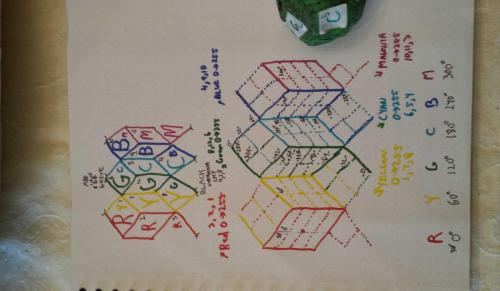Regards, TAR

Edited by tar
##### Share on other sites

• 2 weeks later...

Well the HAS color system is not going to work out. Pigments are too irregular and its difficult...if not impossible...to add color using subtractive pigments. I thought white would help...but doesn't look good.

But, in terms of the 12 segments of the sphere, the 2 dimensional layout proposed in the previous post is nice, and could be applicable to other things. The three points, and the four points are all there, exactly as they are on a spherical rhombic dodecahedron. You have four intersecting hexagonal planes, depicted in 2 dimensions. The grid system seems pretty close to regular, except for the shield shapes at the three points.

In the color system, I divided the center segments vertically up into smaller sections than the top and bottom, because its hard to divide a number like 256 into 3 parts, so that is not very regular, but with the HAS system put aside, there may still be some useful uses of the scheme, which allows the sphere to be thought of in terms of a grid with "almost" regular grid components. You still have four intersecting 360 degree, six section "wheels" that completely cover the sphere, with no overlap.

Nice to have a clean, 2D way to represent it.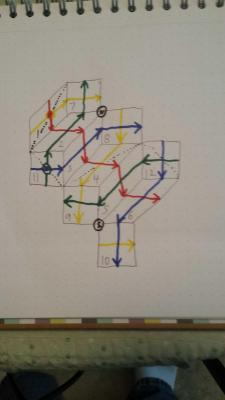Actually I would like to set a convention and put the number 1 segment in the center, with the red hexagonal plane numbers going up and to the right, and the yellowplanes numbers going up and to the left.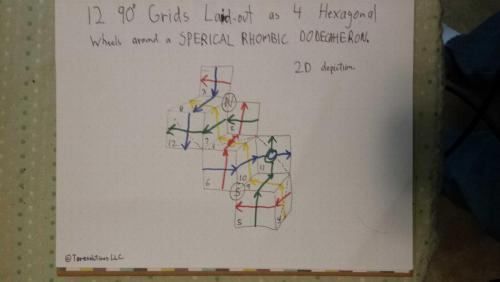Regards, TAR

Edited by tar
##### Share on other sites

Well the HAS idea does not work well, but I am considering working it a little differently, using my original color conventions in the same arrangement. The red six section wheel will show cyan, the yellow will show brightness or saturation (B&W grey scale) the blue will show yellow and the green will show magenta. It might be interesting. I have not tried it yet. If it works I might patent it and sell it to paint stores to display their colors.

But, in preparation, I wrote out how to translate the degrees into percentage, or into a 0 to 255 scale. There may be other applications where 4 variables and their intersections might be interestingly displayed with this scheme.

You have the 12 grids, each 60 degrees by 60 degrees. You can regularly break each grid in half both ways (30 degree divisions) giving you 4x12 or 48 areas, or use 15 degree divisions which breaks each of the 12 sections into a 4x4 grid, giving you 16x12 or 192 sections, or use 20 degree sections yielding 12 3x3 grids or 108 segments, or use 10 degree by 10 degees giving you 12x36 or 432 sections. Or of course 1 degree giving you (60x60x12) or 43200 sections.

Well...anyway for starters here is a worksheet showing how degrees would line up with percentage and a 0-255 scale...if it gives anybody any ideas of visualizing 4 interelated circular scales (variables) at once.

You can go back and forth between degrees and percentage, using 3.6 and .27777.

Between degrees and a 256 scale using 1.40625 and .711111.

And between percentage and 0-255 using .390625 and 2.56.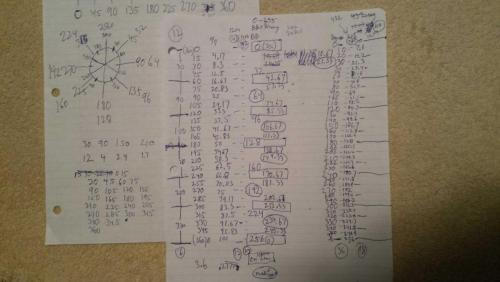Regards, TAR

Edited by tar
##### Share on other sites

Actually the rectangles and ovals are reversed in meaning from the legend in the central column of 0-255 numbers. The ovals are in the center of the segment and the rectangles are on the lines between the six segments of the wheel. You can do it either way, but to be consistent with the original scheme, the 0/360 point or the 0/256 point should be in the center of the segment. (Segment 1 has the 0/256 point of Red and Yellow and segment 11 has the 0/256 point of Blue and Green in the center of the segment)

So the whole worksheet is actually incorrect, as it shows the 0 points on the line between segments and should show for instance segment 1 as running from 330(234.7) to 30(21.3) not 0(0) to 60(42.7). Everything should be rotated back 30 degrees, or 8.3 percent or 21.3 in the case of the 0-255(6) scale.

Edited by tar
##### Share on other sites

• 1 month later...

Back to the 12 section of the sphere, taradian grid concept, using the spherical rhombic dodecahedron layout, with each diamond cut into quarters, and then each quarter cut in quarters to yield 16 numbered sections per diamond, associatable to, or arrived at, by making 15 degree lines on the six segment "wheels" of the red hexagon plane, the yellow hexagonal plane, the blue hexagonal plane and the green hexagonal plane.

Here is the scheme, shown in 2D. The large coin is drawn with color markers that bled through to the other side, but that retained the scheme exactly. All sections appear in their entirety, exactly as they would if you looked at a sphere thusly divided, except for sections 11 an 12, where you see half of both on one side, and the other half of both on the other.

Regards, TAR

(Design copyright TAResolutions 2015)

##### Share on other sites

• 4 weeks later...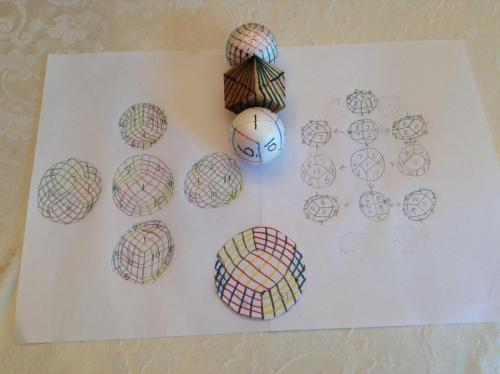The cube in this photo is actually upside down, as the number 4 diamond is "up" and I meant to put the number one diamond up, but the two reasons for the photo are still there. One is to show three two dimensional representations of the 15 degree segmented figure. As you can see a three point in the center of a 2D circle yields three full segments and six half segments. A fourpoint in the center yields 4 full segments and 4 half segments, and a segment in the center yields 5 full segments and 2 half segments.

The representation with the number one segment in the center is rotated slightly to a four-point or a three-point to show the orientation of the various segments to each other.

The other purpose of the picture is to show the green 15 degree grid lines on the cube as if they were extended to the three points that exist on the axis of the green hexagonal "wheel".

It is an interesting attribute of the 15 degree line layout, that the lines are actually all segments of great circles that intersect at the axis point, top and bottom.

The yellow grid line great circles intersect at the 2,3,11 and the 5,6,12 three-points.

The great circles of which the red grid lines are segments intersect at the 7,8,12 and 9,10,11 three-points.

Those of the blue intersect at the 1,2,7 and 4,5,9 three-points, and those of the green, as extended on the cube intersect at the 3,4,8 and the 1,6,10 three points.

The interesting thing, is that even though the grid lines are segments of great circles, which would seem to demand that the lines be "closer" to each other, as you near the three-points and similarly the farthest away from each other at the midpoint, between the two three points, it appears on the cube, that the green grid lines, that are within the diamonds of the green hexagonal wheel, are evenly spaced in reference to each other.

Regards, TAR

##### Share on other sites

After all that, I find I have the two coins with the three point at the center, drawn wrong. I will redo, but the 6 half segments are drawn wrong. There should be 2+ grids showing on one end of each of the partial segments and 1+ grids showing on the other end of each of the partial segments, which I have, but I have it reversed from how it should be. The 2+ grids should be along the line coming from the center, after a four point. The 1+ grid lines should show on the line coming from a three point near the edge.

Sorry. Sort of bad to get my own 2d depiction wrong.

Here is the right way.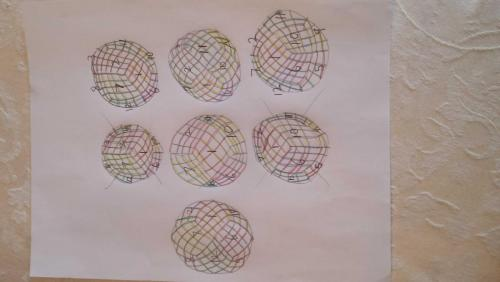Regards, TAR

Edited by tar
##### Share on other sites

• 2 weeks later...

Earlier in the thread I speculated that the length of the edge of each diamond of the spherical rhombic dodecahedron, along the surface of the sphere, was either a radius long or 1/6 of a circumference long, I couldn't tell. I now don't think it is either.

Using a great circle mapper / distance calculator (http://www.gcmap.com/mapui?P=0%B0N+90%B0E-35%B023%27N+45%B0E&MS=wls&DU=mi) I found that the three-points of the figure, when placed on the globe, would have equal length sides if they were at approximately 35 degrees 23 minutes north and south. The four points are on the equator 45 degrees East and West of a three-point's longitude. That way the distance from the three point to the pole is 3781 miles and the distance from the three-point to the point on the equator 45 degrees longitude away, was 3782 miles.

My original thinking was that the three points would be at 45 degrees latitude, but the calculator using those locations says the distance from the three point to the pole would be 3117miles and the distance from the three point to the equator point would be 4140. So the 35 degree 23 minute positioning seems to be the correct positioning to make each side of each of the 12 diamonds, the same length.

The circumference of the Earth is 24,901.55 around the equator and 24,859.82 through the poles. Using the equator figure a 1/6 of this would be 4150 miles. Not 3781 miles.

The circumference of 24,901 divided by pi would give you the diameter of 7926. Half of this is 3963, which is also not 3781 miles.

Interestingly 3963/3781 equals 1.048 and 1/3 pi is 1.047. So the length of the segment of the great circle that is coincident with the diamond's edge is .955 that of the radius of the sphere.

Thus the dimensions of the spherical rhombic dodecahedron diamond, when place over the globe, are approximately 3781 miles per side, with the short diagonal at about 4889 miles (24901 minus 4 times 3781 leaving 9777, which divided by 2 is 4889) and the long diagonals at around 6225 (1/4 circumference of 24900.)

Regards, TAR

##### Share on other sites

The twelve segments look like this, when imposed on the globe, using the poles the equator and the 35 degree 23 minute latitude lines, North and South. The spacing of the equatorial four points is 90 degrees, and the spacing of the north and south three-points is 90 degrees, and there is 45 degrees between the three points and the equatorial four points.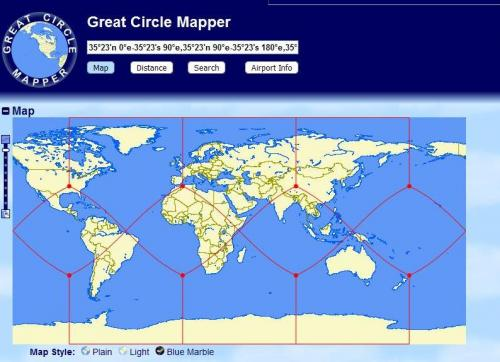Regards, TAR

##### Share on other sites

The twelve segments look like this, when imposed on the globe, using the poles the equator and the 35 degree 23 minute latitude lines, North and South. The spacing of the equatorial four points is 90 degrees, and the spacing of the north and south three-points is 90 degrees, and there is 45 degrees between the three points and the equatorial four points.mapper2.JPG

Regards, TAR

.

.

Very clever, and very impressive , I must say . I think your name is going to go down in history as :-

. . . " The Tar Projection" . . .

Mike

Edited by Mike Smith Cosmos
##### Share on other sites

• 2 months later...

!

Moderator Note

Hijack regarding real vs ideal nature of spheres split off to new thread

Fred Champion - from memory you have been asked before not to interrupt threads with your own speculations and new ideas; instead open a new thread (you can include a link to the old thread that prompted your branch in the OP. Do NOT just go off at a tangent and post new ideas and supposition

No need to respond to this modnote - report if you think it is unfair

##### Share on other sites

• 4 weeks later...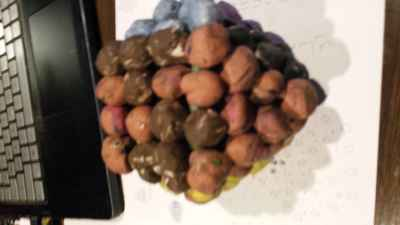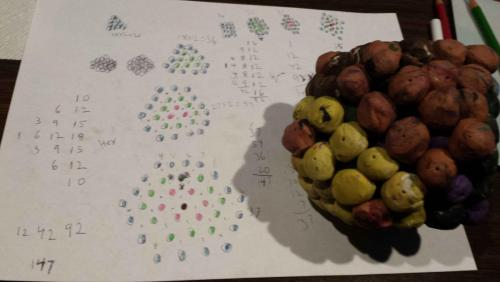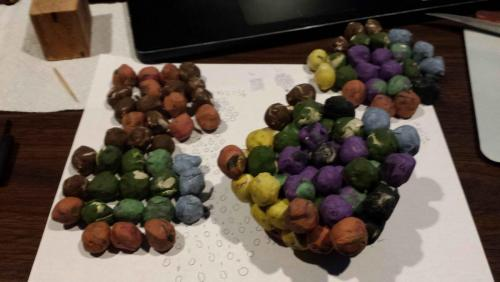After just building the figure out in clay to the third level, like Janus rendered early on in the thread, I counted up the balls required on each level and took it apart layer by layer to see what each of the hexagonal planes and each of the square planes looked like. The buildout is in the shape of a cubic octahedron. As each layer is added it retains this feature. There are 3 distinct square stacks and 4 distinct hexagonal stacks, that is you can see the figure as a stack of 7 square planes 3 different ways and a stack of seven hexagonal planes 4 different ways...at the same time.

Where I took it apart and laid out three of the 7 square plane segments the one at 1 o'clock would go back on first, then the one at 8 o'clock and then the one at 10.

Regards, TAR

## Create an account or sign in to comment

You need to be a member in order to leave a comment

## Create an account

Sign up for a new account in our community. It's easy!

Register a new account